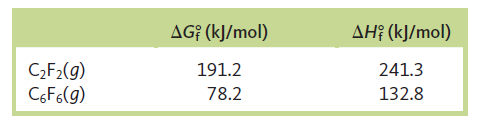# Problem: The standard free energies of formation and the standard enthalpies of formation at 298 K for difluoroacetylene (C2F2) and hexafluorobenzene (C6F6) areFor the following reaction:                C  6F6 (g) ⇌ 3C 2F2 (g)a. calculate ΔS° at 298 K.

###### FREE Expert Solution

C6F6 (g) ⇌ 3 C2F2(g)

$\overline{){\mathbf{\Delta G}}{{\mathbf{°}}}_{{\mathbf{rxn}}}{\mathbf{=}}{\mathbf{\Delta H}}{{\mathbf{°}}}_{{\mathbf{rxn}}}{\mathbf{-}}{\mathbf{T\Delta S}}{{\mathbf{°}}}_{{\mathbf{rxn}}}}$

Step 1: We can use the following equation to solve for ΔH˚rxn:

ΔH˚rxn = 591100 J/mol

Step 2: We can use the following equation to solve for ΔG˚rxn:

ΔG˚rxn = 495400 J/mol

Step 3: Calculate for ΔS˚rxn

90% (153 ratings)###### Problem Details

The standard free energies of formation and the standard enthalpies of formation at 298 K for difluoroacetylene (C2F2) and hexafluorobenzene (C6F6) areFor the following reaction:
C  6F6 (g) ⇌ 3C 2F2 (g)

a. calculate ΔS° at 298 K.

Frequently Asked Questions

What scientific concept do you need to know in order to solve this problem?

Our tutors have indicated that to solve this problem you will need to apply the Gibbs Free Energy concept. You can view video lessons to learn Gibbs Free Energy. Or if you need more Gibbs Free Energy practice, you can also practice Gibbs Free Energy practice problems.

What professor is this problem relevant for?

Based on our data, we think this problem is relevant for Professor Nair's class at TAMU.

What textbook is this problem found in?

Our data indicates that this problem or a close variation was asked in Chemistry: An Atoms First Approach - Zumdahl Atoms 1st 2nd Edition. You can also practice Chemistry: An Atoms First Approach - Zumdahl Atoms 1st 2nd Edition practice problems.